Like   Tweet   Pin   +1   in

# Hi ,

Happy Halloween from Ancient Wisdom Team. We hope you have had a great Halloween day so far. We are giving away £10+VAT Voucher on your next order. Simply copy and paste or write the code Halloween2018 into your shopping cart and press add voucher and we will automatically deduct £10 off your order.

Voucher code valid until 7th of November at 5pm.

 /* styles */ Hurry and get ready...I can feel this year's Halloween is going to be extra scary. Rodrigo£10 off on your next order. Voucher code: Halloween2018
 /* styles */ We need your help to judge the winner of the best costume, see below. If you vote by replying to this email by 2nd of November by 5pm you will get a FREE Box of Winter Berry Soy Pot of Candles, at £17.70 at retail price.FREE - Box Winter Berry Soy Pot of Fragrance Candles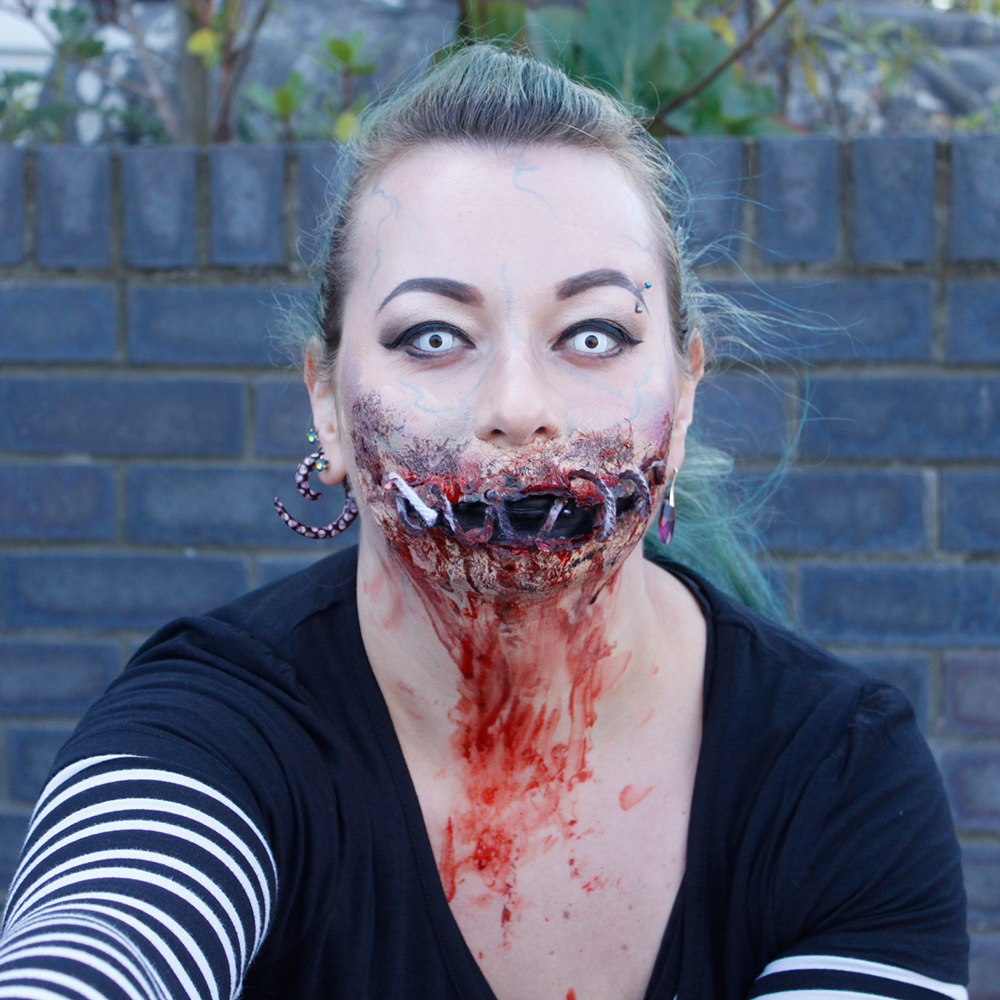Urszula - Email OnlyJacob - Judge Dredd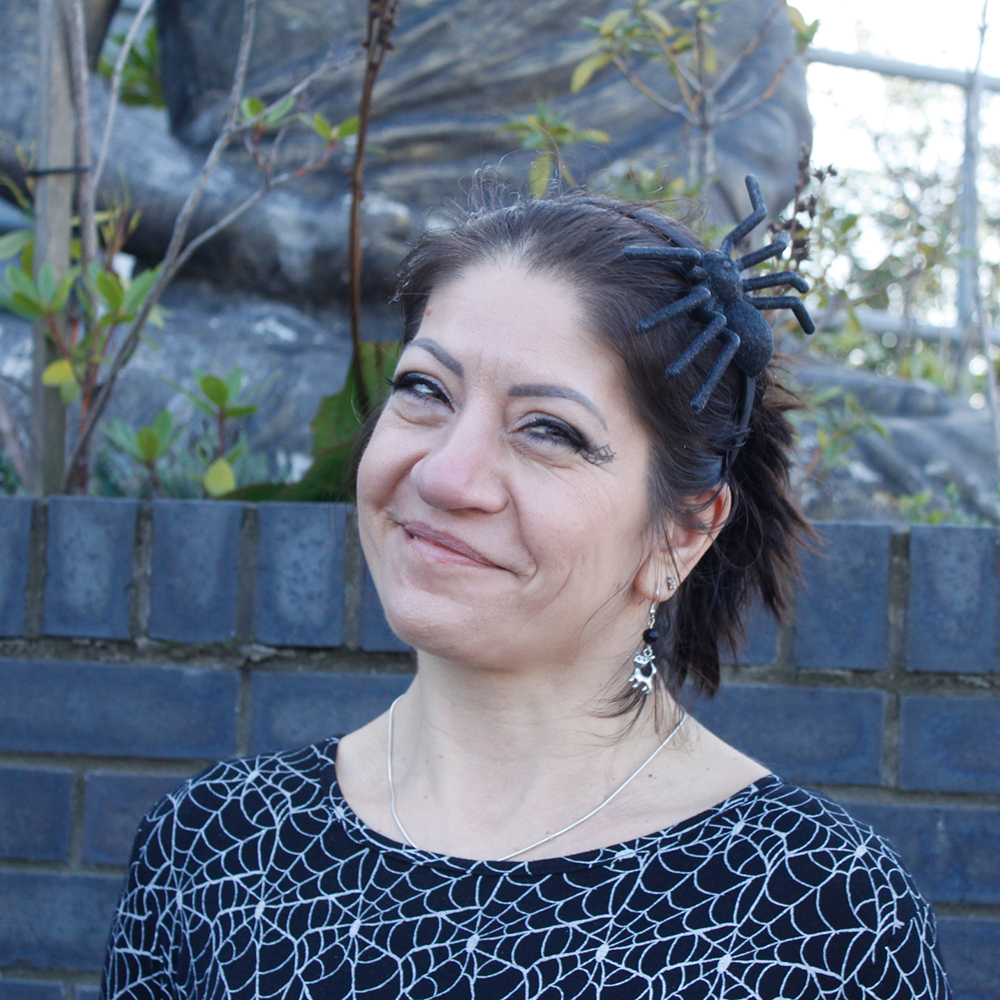Joanna - Spider Lady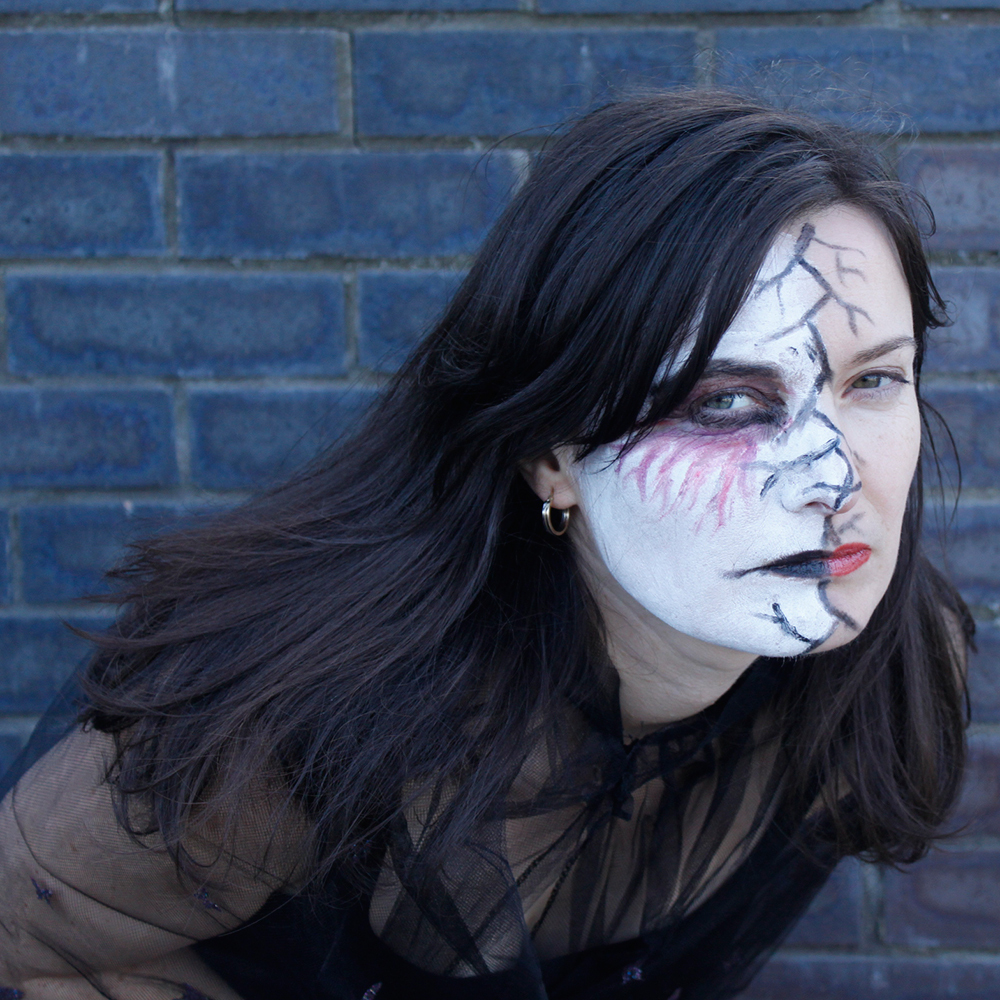Breaking Bad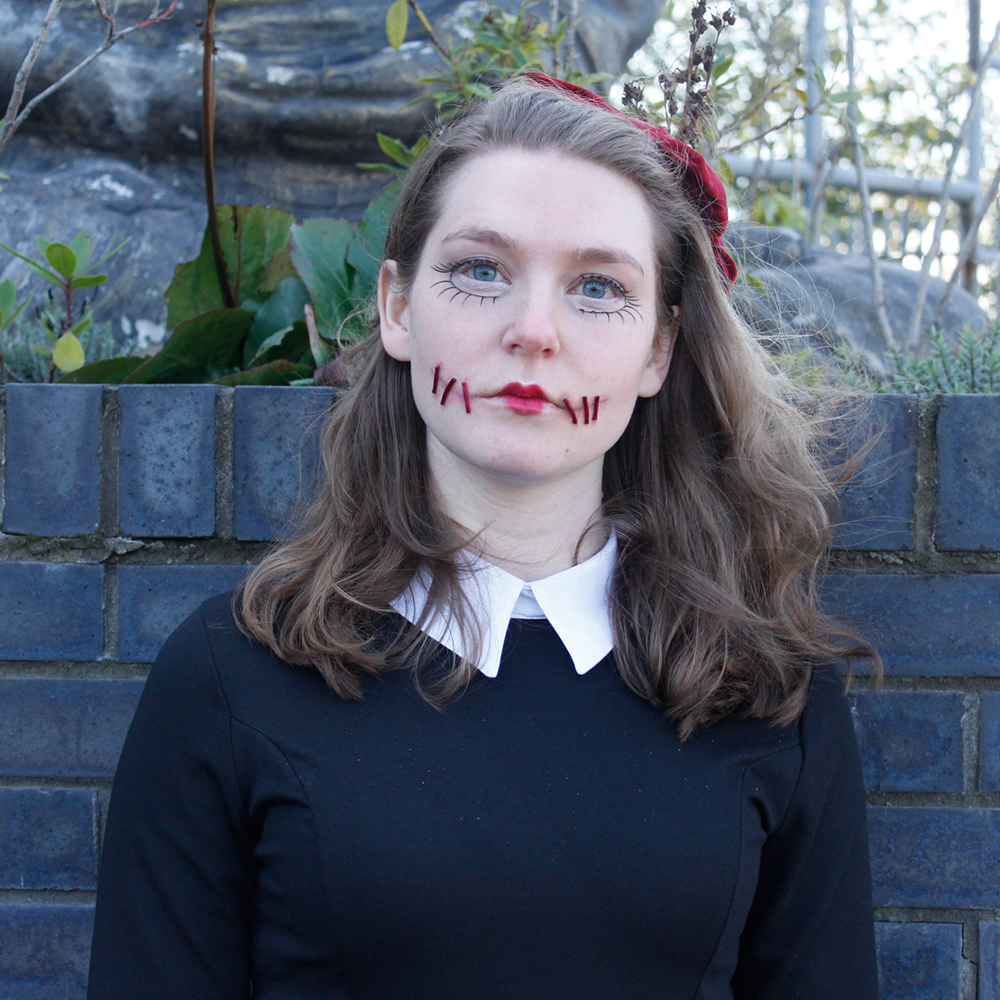Kristy - The Haunted Doll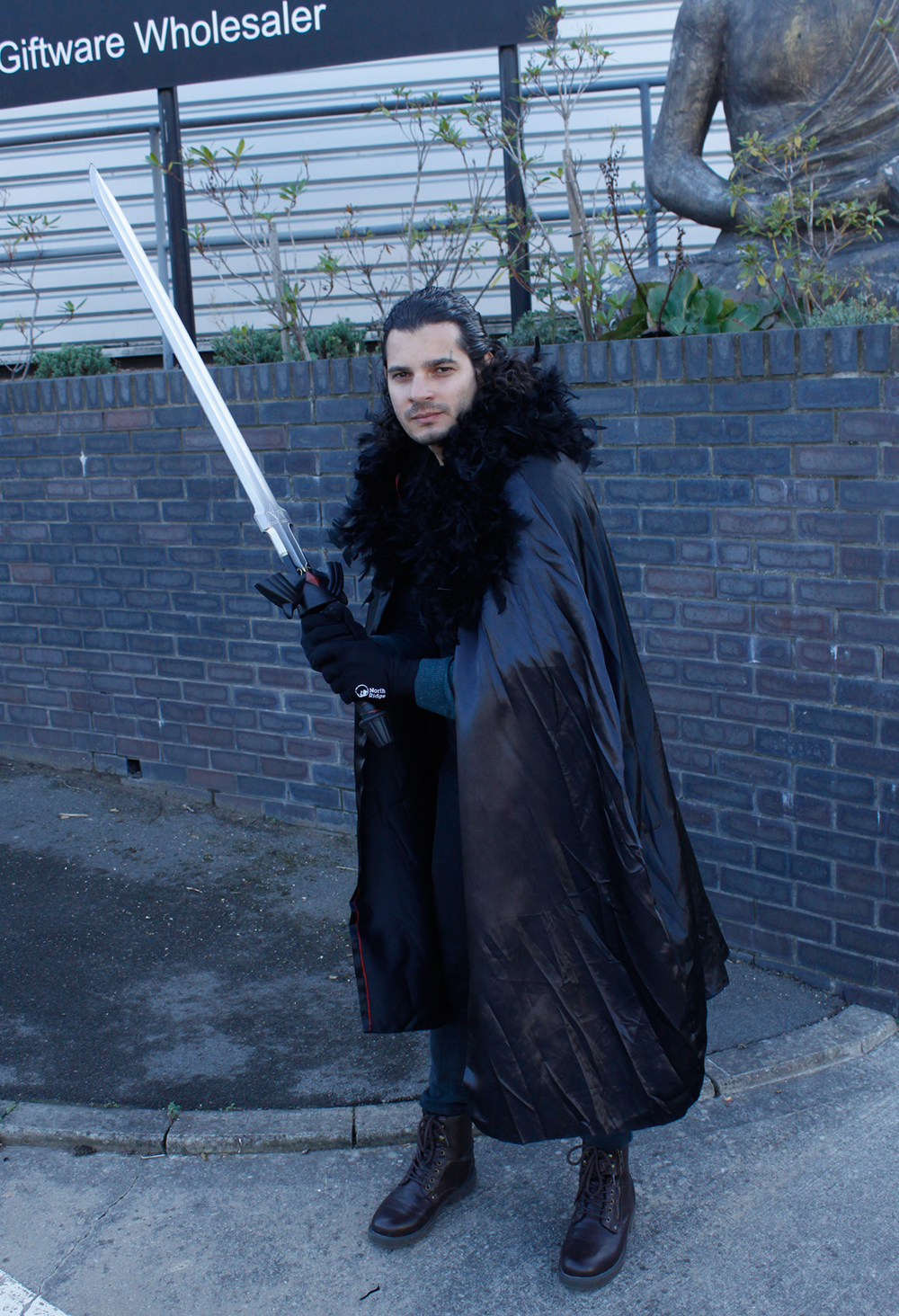The Winter is Coming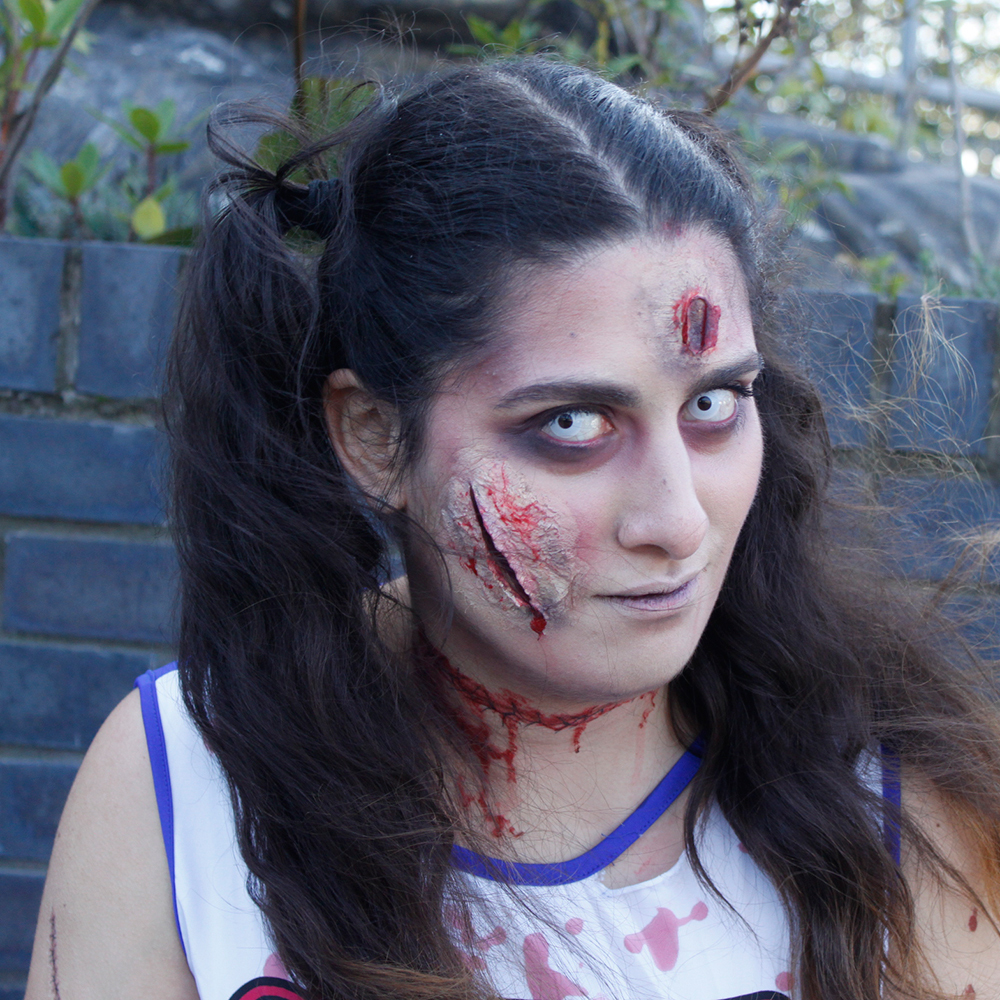Tiphanie Cheeleader ZombieJonathan: Caerbannog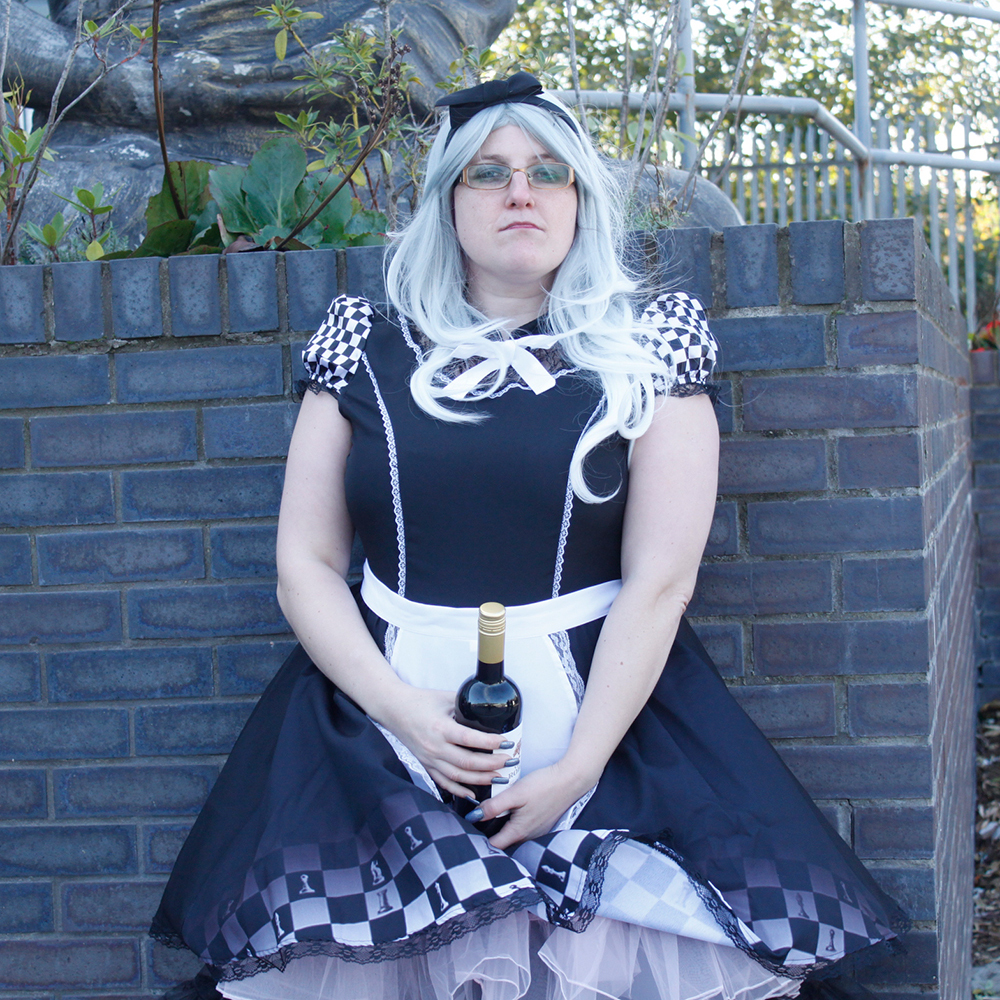Kerry - Dark Alice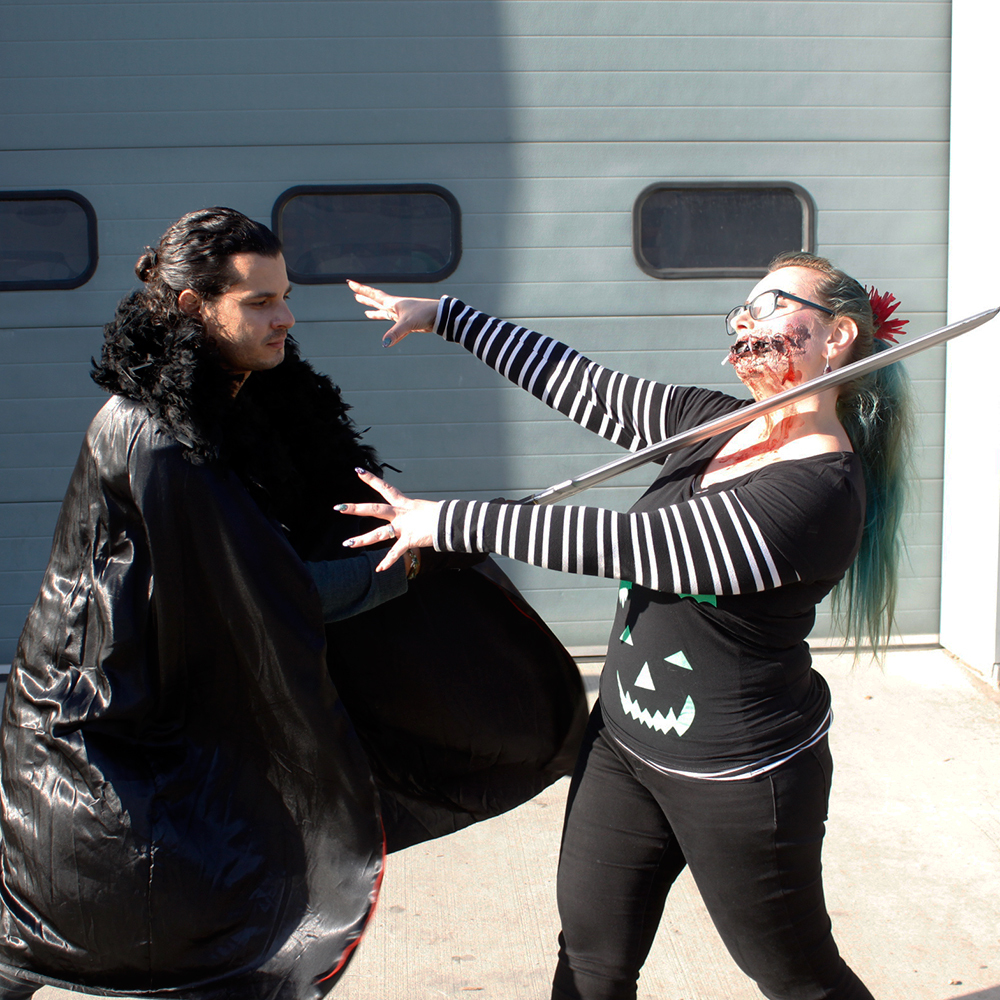Winter is coming Vs email only
 table div table+table+table+table+table+table+table+table+table+table+table+table+table div table{width:100%;padding:0}table div table+table+table+table+table+table+table+table+table+table+table+table+table div table table{padding:0;float:left!important;width:51.886%!important}table div table+table+table+table+table+table+table+table+table+table+table+table+table div table table+table td,table div table+table+table+table+table+table+table+table+table+table+table+table+table div table td{padding-left:0;padding-right:0}table div table+table+table+table+table+table+table+table+table+table+table+table+table div table table td{padding-left:0;padding-right:20px}table div table+table+table+table+table+table+table+table+table+table+table+table+table div table table+table{float:left!important;width:48.114%!important}/* styles */
 table.module-13{width:99.25%;padding:0}table div table+table+table+table+table+table+table+table+table+table+table+table+table+table div table{width:99.25%;float:none;margin-left:auto;margin-right:auto;padding:0}table div table+table+table+table+table+table+table+table+table+table+table+table+table+table div table a{border:0 none;text-decoration:none}table div table+table+table+table+table+table+table+table+table+table+table+table+table+table div table img{width:100%!important;border:0 none;text-decoration:none}table div table+table+table+table+table+table+table+table+table+table+table+table+table+table div table td{width:100%;padding:0}/* styles */
 table div table+table+table+table+table+table+table+table+table+table+table+table+table+table+table div table td,table.module-14{width:100%;padding:0}table div table+table+table+table+table+table+table+table+table+table+table+table+table+table+table div table{width:100%;float:none;margin-left:auto;margin-right:auto;padding:0}table div table+table+table+table+table+table+table+table+table+table+table+table+table+table+table div table a{border:0 none;text-decoration:none}table div table+table+table+table+table+table+table+table+table+table+table+table+table+table+table div table img{width:100%!important;border:0 none;text-decoration:none}/* styles */